# Maths - Morphisms Between Topologies

The most important part of this subject is the relationship between different topologies. The mappings or morphisms between them.

 For example, the continuous 'rubber geometry' deformations as between a toroid and a mug.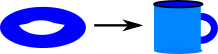There are two related mapping types continuous mappings and homeomorphisms, we start with continuous mappings.

 One condition for a continuous mapping is that the intersections and unions of neighbourhoods play together properly.This can be shown in terms of logic like this:Or we can show it in terms of a simplex:We can see, for instance, that if there is a meet (A/\B) in the domain but not the codomain this implies a sort of tear. Where to elements of A/\B map to?### Open Set Criterion for Continuity

A map ƒ: M1 -> M2 is continuous if and only if the inverse image of every open set is open.

#### Definition 1

Here is one definition of continuity, based on open sets:

 Let X and Y be topological spaces. A function f : X->Y is continuous if f-1(V) is open for every open set V in Y.#### Definition 2

Another definition of continuity, based on neighbourhoods, is equivalent to the above definition.

 Let X and Y be topological spaces. A function f : X->Y is continuous if for every xX and every open set U containing f(x), there exists a neighbourhood V of x such that f(V)U.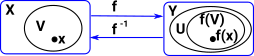More detail about continuity on page here.

### What does a continuous mapping look like?

 A continuous mapping may collapse multiple points to single points.Here we convert that to neigbourhoods.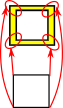So this looks like a fibre bundle as discussed on the page here.

### Counter Examples### Examples - Möbius Band to Circle

 Here we have a morphism which collapses the width of the Möbius band.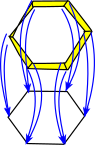when we reverse this morphism we keep the global structure and loose some local structure. We also loose the twist in the Möbius band.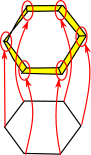### Examples - Helix to Circle

 Here we have a morphism which collapses a helix to a circle.### Homeomorphisms

 A homeomorphism preserves 'nearness' but allows 'rubber geometry' deformations. Note: homeomorphism is a different concept from homomorphism.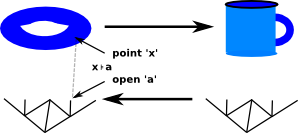### Projections

 We can project helix onto circle and still keep continuity.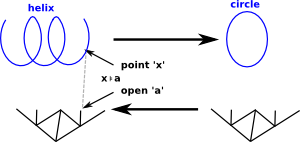metadata block see also: Correspondence about this page Book Shop - Further reading . Where I can, I have put links to Amazon for books that are relevant to the subject, click on the appropriate country flag to get more details of the book or to buy it from them.Introduction to Topological Manifolds (Graduate Texts in Mathematics S.) Other Books about Curves and Surfaces

This site may have errors. Don't use for critical systems.